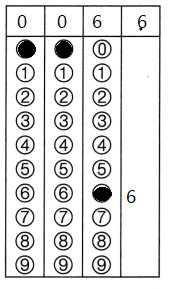Refer to our Texas Go Math Grade 3 Answer Key Pdf to score good marks in the exams. Test yourself by practicing the problems from Texas Go Math Grade 3 Module 13 Assessment Answer Key.

Concepts and Skills

Question 1.
Explain how to find 30 ÷ 6 by making equal groups. TEKS 3.4.H
Explanation:
5 + 5 + 5 + 5 + 5 + 5 = 30
5 is divided in to 6 groups

Find the quotient. TEKS 3.4.F, 3.4.J

Question 2.
12 ÷ 6 = ___
Explanation:
6 x 2 = 12
Quotient = 2

Question 3.
___ = 48 ÷ 6
Explanation:
6 x 8 = 48
Quotient = 8

Question 4.
63 ÷ 7 = ___
Explanation:
Quotient = 9
9 x 7 = 63

Question 5.
___ = 27 ÷ 9
Explanation:
Quotient = 3
9 x 3 = 27

Question 6.Explanation:
Quotient = 7
7 x 7 = 49

Question 7.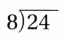Explanation:Quotient = 4
8 x 4 = 24

Question 8.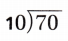Explanation: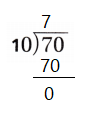Quotient = 7
10 x 7 = 70

Question 9.Explanation:Quotient = 8
9 x 8 = 72

Question 10.Explanation: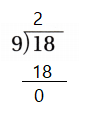Quotient = 2
9 x 2 = 18

Question 11.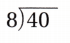Explanation:Quotient = 5
8 x 5 = 40

Question 12.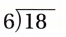Explanation: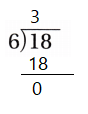Quotient = 3
6 x 3 = 18

Question 13.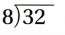Explanation:Quotient = 4
8 x 4 = 32

Find the unknown factor and quotient. TEKS 3.4.F, 3.4.J

Question 14.
6 × ___ = 42 42 ÷ 6 = ___
6 × 7 = 42
42 ÷ 6 = 7
Explanation:
Found the unknown factors
and quotient = 7
7 x 6 = 42

Question 15.
7 × __ = 28 ___ = 28 ÷ 7
7 × 4  = 28
4 = 28 ÷ 7
Explanation:
Found the unknown factors
and quotient = 4

Question 16.
__ × 8 = 16 16 ÷ 8 = ___
2 × 8 = 16
16 ÷ 8 = 2
Explanation:
Found the unknown factors
and quotient = 2

Question 17.
6 × __ = 60 __ = 60 ÷ 6
6 × 10 = 60
10 = 60 ÷ 6
Explanation:
Found the unknown factors
and quotient = 10

Texas test Prep

Fill in the bubble for the correct answer choice.

Use strategies to solve.

Question 18.
Maria has 54 flower seeds and 9 pots. If she wants to put an equal number of seeds in each pot, how many seeds will Maria put in each pot? TEKS 3.4.F, 3.4.J, 3.4.K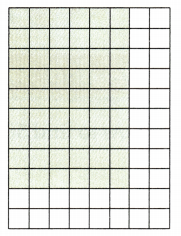(A) 6
(B) 7
(C) 8
(D) 9
Explanation:
6 seeds will Maria put in each pot

Question 19.
There are 56 students at basketball camp. The coaches put 8 players on each team. How many teams did they make? TEKS 3.4.F, 3.4.K, 3.5.B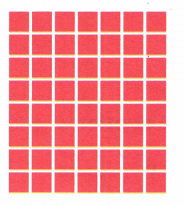(A) 6
(B) 7
(C) 48
(D) 64
Explanation:
8 x 7 = 56
The coach makes 7 teams

Question 20.
Tristan bought 14 tickets when he arrived at the fair. Then he bought an additional 7 tickets to go on his favorite ride. The ride costs 7 tickets. How many times can Tristan go on his favorite ride? TEKS 3.4.F, 3.4.J, 3.4.K
(A) 24
(B) 18
(C) 9
(D) 3
Explanation:
3 times can Tristan go on his favorite ride
14 tickets when he arrived at the fair
additional 7 tickets to go on his favorite
14 + 7 = 21
21 divided by 7 = 3

Question 21.
Liza bought 48 inches of ribbon to make bows. If she uses 8 inches of ribbon for each bow, how many bows can she make? TEKS 3.4.F, 3.4.J, 3.4.K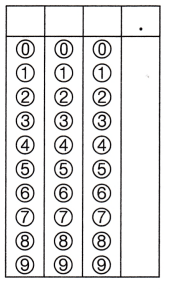Record your answer and fill in the bubbles on the grid. Be sure to use the correct place value.